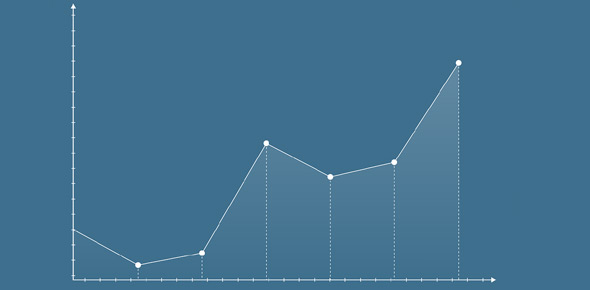2614 Scatter Plot Transformations Logs

15 Questions | Total Attempts: 268SettingsLog x and log y transformations

• 1.
The log y transformation linearises the scatter plot by :
• A.

Turning the y values into log y values

• B.

Compressing the y axis values

• C.

Stretching the y axis values

• D.

Leaving the x axis values the same

• 2.
The log x transformation linearises the scatter plot by :
• A.

Changing the x values to log x values

• B.

Compressing the x axis values

• C.

Stretching the x axis values

• D.

Leaving the y values as they are

• 3.
The scatter plot above will potentially be linearised with :
• A.

Log x transformation

• B.

Log y transformation

• C.

It is is the wrong quadrant for these transformations

• 4.
The scatter plot above will potentially be linearised with :
• A.

Log x transformation

• B.

Log y transformation

• C.

It is is the wrong quadrant for these transformations

• 5.
The scatter plot above will potentially be linearised with :
• A.

Log x transformation

• B.

Log y transformation

• C.

It is is the wrong quadrant for these transformations

• 6.
The scatter plot above will potentially be linearised with :
• A.

Log x transformation

• B.

Log y transformation

• C.

It is is the wrong quadrant for these transformations

• 7.
Which quadrants have potential for a log x transformation
• A.

• B.

• C.

• D.

• 8.
Which quadrants have potential for a log y transformation
• A.

• B.

• C.

• D.

• 9.
The residual plot above shows the original data is potentially from which quadrants
• A.

• B.

• C.

• D.

• 10.
The residual plot above shows the original data is potentially from which quadrants
• A.

• B.

• C.

• D.

• 11.
The residual plot above shows the original data can potentially be linearised with :
• A.

Log x transformation

• B.

Log y transformation

• C.

It can't be linearised with a log transformation

• 12.
The residual plot above shows the original data can potentially be linearised with :
• A.

Log x transformation

• B.

Log y transformation

• C.

It can't be linearised with a log transformation

• 13.
The residual plot above shows the original data can potentially be linearised with :
• A.

X squared transformation

• B.

Y squared transformation

• C.

It can't be linearised with a log transformation

• 14.
The log transformations are not applicable in which quadrant
• A.

• B.

• C.

• D.

• E.

• 15.
The image above is a transformer in Bubble Bee form from :
• A.

• B.

• C.Back to top